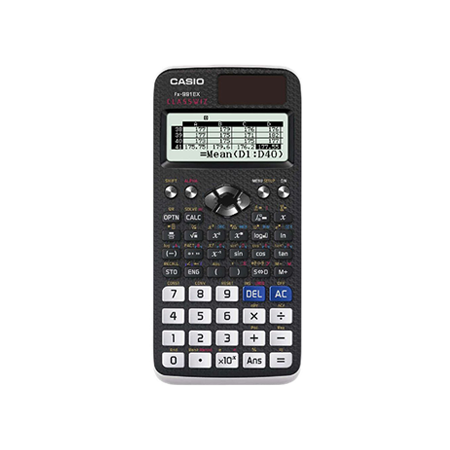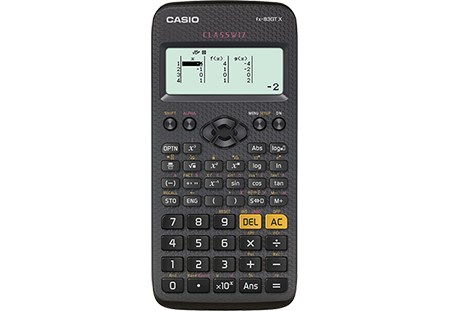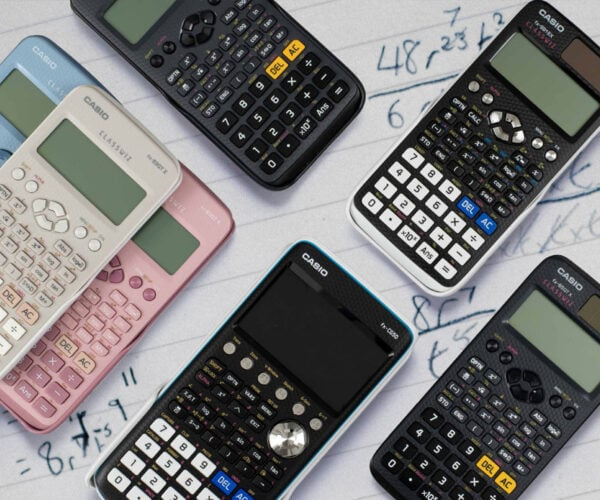Scientific Calculators - Casio Calculators
Unmatched Possibilities

The FX-991 is our most advanced scientific calculator approved by exam boards in the UK market.

## Scientific Calculators

Students will need a scientific calculator for GCSE Maths. Scientific calculators handle standard arithmetic, and then add extra functionality like cube roots, powers, statistics, trigonometry and more. They are also very useful for other subjects such as Science, so one model covers all your subjects.

A basic scientific calculator is the minimum you require for exams. However a more advanced model such as the fx-991EX will give you advantages for learning and in the exam, for example with solving equations.fx-991EX

A-Level Further Maths GCSE

The Casio fx-991EX is Casio’s most advanced scientific calculator. Part of the ClassWiz range – features include calculus, probability distributions, equation solvers, matrices and vectors, and spreadsheets.fx-85GTX

A-Level Further Maths GCSE IB Ireland JC & LC

### Not all scientific calculators are created equal

ClassWiz technology in the fx-85GTX significantly importunes ease of use. The high resolution menu-driven display is crisp, with scrolling results and tables.fx-83GTX

A-Level Further Maths GCSE IB Ireland JC & LC

### The Simplistic Approach To Equations

Our entry level ClassWiz Scientific Calculator covers all the mathematical requirements for first
examinations.Maths is a visual subject. A graphic calculator allows you to draw graphs and statistical charts, while doing the same calculations as on a scientific calculator. Some schools now use our fx-CG50 graphic calculator which gives a significant learning advantage, especially at the Higher tier. Please see below on why it might be worth considering this calculator.### Resources

Casio offer a large suite of resources available for UK schools and cover teaching with calculators alongside the most popular subject topics for GCSE and A Level.### Emulators

Teachers can request a free licence for use in classrooms, remote and blended learning (available in the UK, Channel Islands, Isle of Man and Ireland).### Comparison Chart

There are two main types of calculator: scientific and graphic. A scientific calculator can only be used for calculation. A graphic calculator makes calculations, shows surds as exact answers and displays graphs.